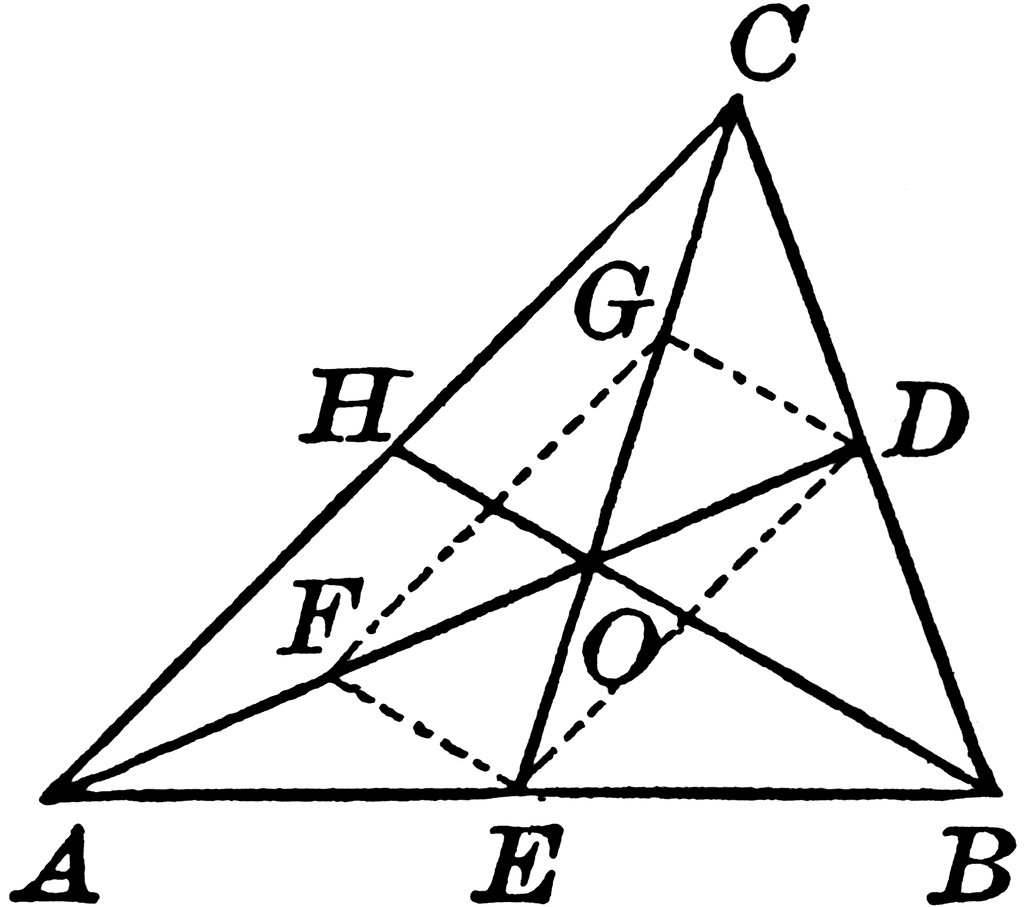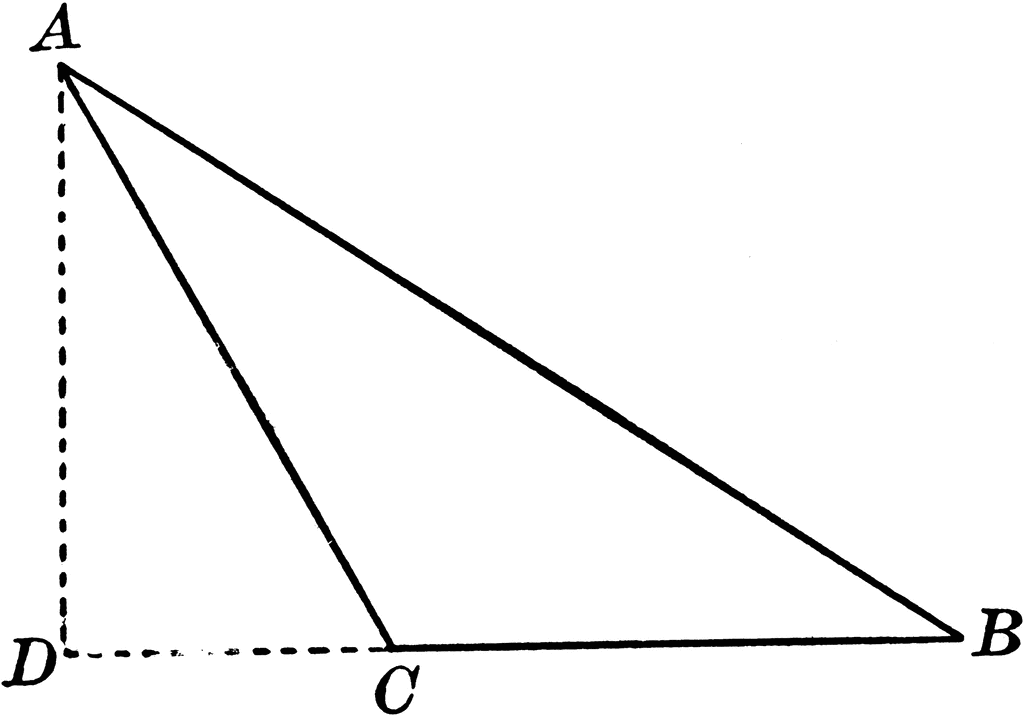# Difference between Altitude and Median

A triangle is a plane figure with three straight sides and three angles. It contains several different components which includes median and altitude. Apart from these two components it has a vertex, a base, area, perimeter, interior and exterior angles. In order to know about a triangle and all its properties, one must learn to calculate all these different components.

The basic difference between a median of a triangle and its altitude is relatively basic. The median of a triangle is the line which is joining up with the vertex and stretching to the midpoint of the opposite side. On the other hand, the altitude is the straight line which passes through the vertex and the perpendicular towards the other side or extends to the other side, opposite to the first vertex.

Another difference between these two is the way they are calculated. In order to calculate the median of the triangle, one must first draw the triangle on graph paper. It helps you calculate the midpoint between two points, or a vertex which is on the opposite side. Once you know the two points, it is easy to calculate their midpoint, which will eventually be the median. On the other hand, the altitude is extremely easy to calculate. Once you have drawn the triangle on a graph paper, you can simply calculate the distance between the two points which are falling under the category of altitude.

### Instructions

• 1

Median

The median of a triangle is the midpoint between the vertex of the two opposite sides. In total, there are three vertices, which mean that there are three possible medians. An interesting fact to note about these vertices is that no matter which shape the triangle is, once you sketch a line from these means to the opposite side, they will all join together at one point, which is known as the centroid. The median of a triangle has certain properties. First, the three medians must meet at a single point. Secondly, the triangle is divided into smaller triangles by the median.

- Image Courtesy: etc.usf.edu• 2

Altitude

The altitude is also the perpendicular that is stretched from the base to the opposite vertex of the triangle. A triangle basically has three possible bases that can give three different altitudes. These three altitudes, once stretched from their origin, all join together at a single point called the orthocenter.

- Image Courtesy: etc.usf.edu•